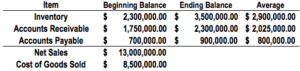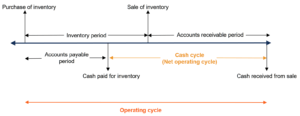# Operating Cycle

The number of days required to receive, sell and receive cash from the sale of inventory

## What is an Operating Cycle?

An Operating Cycle (OC) refers to the days required for a business to receive inventory, sell the inventory, and collect cash from the sale of the inventory. This cycle plays a major role in determining the efficiency of a business.### Formula

The OC formula is as follows:

##### Operating Cycle  =  Inventory Period + Accounts Receivable Period

Where:

• Inventory Period is the amount of time inventory sits in storage until sold.
• Accounts Receivable Period is the time it takes to collect cash from the sale of the inventory.

### Uses of the Operating Cycle Formula

Using the Operating Cycle formula above:

• The Inventory Period is calculated as follows:
##### Inventory Period  =  365 / Inventory Turnover

Where the formula for Inventory Turnover is:

##### Inventory Turnover  =  Cost of Goods Sold / Average Inventory

• The Accounts Receivable Period is calculated as follows:
##### Accounts Receivable Period  =  365 / Receivables Turnover

Where the formula for Receivables Turnover is:

##### Receivables Turnover = Credit Sales / Average Accounts Receivable

Therefore, the detailed formula for OC is:### Sample CalculationCalculating the OC with the data provided above:

• Inventory Turnover: \$8,500,000 / \$2,900,000 = 2.931
• Inventory Period: 365 / 2.931 = 124.53
• Receivables Turnover: \$13,000,000 / \$2,025,000 = 6.419
• Accounts Receivable Period: 365 / 6.419 = 56.862

Operating Cycle = 124.53 + 56.862 = 181.38 = 182 days

### Importance of the Operating Cycle

The OC offers an insight into a company’s operating efficiency. A shorter cycle is preferred and indicates a more efficient and successful business. A shorter cycle indicates that a company is able to recover its inventory investment quickly and possesses enough cash to meet obligations. If a company’s OC is long, it can create cash flow problems.

A company can reduce its OC in two ways:

1. Speed up the sale of its inventory: If a company is able to quickly sell its inventory, the OC should decrease.
2. Reduce the time needed to collect receivables: If a company is able to quickly collect credit sales more quickly, the OC would decrease.

### Net Operating Cycle (Cash Cycle) vs Operating Cycle

The operating cycle (OC) is often confused with the net operating cycle (NOC). The NOC is also known as the cash conversion cycle or cash cycle and indicates how long it takes a company to collect cash from the sale of inventory. To differentiate the two:

• Operating Cycle: The length of time between the purchase of inventory and the cash collected from the sale of inventory.
• Net Operating Cycle: The length of time between paying for inventory and the cash collected from the sale of inventory.

Additionally, the formula for the NOC is as follows:

##### Net Operating Cycle  =  Inventory Period + Accounts Receivable Period –  Accounts Payable Period

The difference between the two formulas lies in NOC subtracting the accounts payable period. This is done because the NOC is only concerned with the time between paying for inventory to the cash collected from the sale of inventory.

The following image illustrates the difference between the two cycles:### Other Resources

CFI’s mission is to help anyone in the world become a great financial analyst through the Financial Modeling & Valuation Analyst designation. To continue learning and advancing your career, these free CFI resources will be helpful: